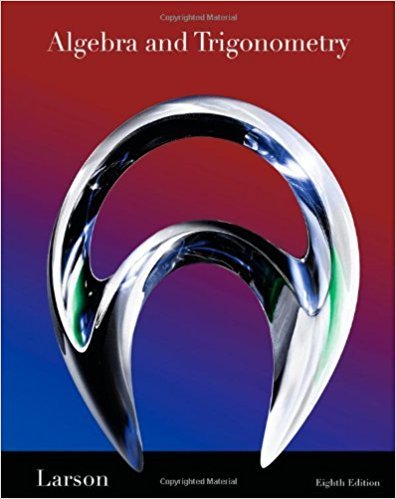×
×

# Solutions for Chapter 10: Matrices and Determinants## Full solutions for Algebra and Trigonometry | 8th Edition

ISBN: 9781439048474Solutions for Chapter 10: Matrices and Determinants

Solutions for Chapter 10
4 5 0 383 Reviews
27
1
##### ISBN: 9781439048474

This textbook survival guide was created for the textbook: Algebra and Trigonometry, edition: 8. Since 154 problems in chapter 10: Matrices and Determinants have been answered, more than 52020 students have viewed full step-by-step solutions from this chapter. This expansive textbook survival guide covers the following chapters and their solutions. Chapter 10: Matrices and Determinants includes 154 full step-by-step solutions. Algebra and Trigonometry was written by and is associated to the ISBN: 9781439048474.

Key Math Terms and definitions covered in this textbook
• Circulant matrix C.

Constant diagonals wrap around as in cyclic shift S. Every circulant is Col + CIS + ... + Cn_lSn - l . Cx = convolution c * x. Eigenvectors in F.

• Cofactor Cij.

Remove row i and column j; multiply the determinant by (-I)i + j •

• Companion matrix.

Put CI, ... ,Cn in row n and put n - 1 ones just above the main diagonal. Then det(A - AI) = ±(CI + c2A + C3A 2 + .•. + cnA n-l - An).

• Cramer's Rule for Ax = b.

B j has b replacing column j of A; x j = det B j I det A

• Diagonal matrix D.

dij = 0 if i #- j. Block-diagonal: zero outside square blocks Du.

• Exponential eAt = I + At + (At)2 12! + ...

has derivative AeAt; eAt u(O) solves u' = Au.

• Fibonacci numbers

0,1,1,2,3,5, ... satisfy Fn = Fn-l + Fn- 2 = (A7 -A~)I()q -A2). Growth rate Al = (1 + .J5) 12 is the largest eigenvalue of the Fibonacci matrix [ } A].

• Free columns of A.

Columns without pivots; these are combinations of earlier columns.

• Hermitian matrix A H = AT = A.

Complex analog a j i = aU of a symmetric matrix.

• Left inverse A+.

If A has full column rank n, then A+ = (AT A)-I AT has A+ A = In.

• Markov matrix M.

All mij > 0 and each column sum is 1. Largest eigenvalue A = 1. If mij > 0, the columns of Mk approach the steady state eigenvector M s = s > O.

• Rank one matrix A = uvT f=. O.

Column and row spaces = lines cu and cv.

• Rank r (A)

= number of pivots = dimension of column space = dimension of row space.

• Schur complement S, D - C A -} B.

Appears in block elimination on [~ g ].

• Semidefinite matrix A.

(Positive) semidefinite: all x T Ax > 0, all A > 0; A = any RT R.

• Subspace S of V.

Any vector space inside V, including V and Z = {zero vector only}.

• Symmetric matrix A.

The transpose is AT = A, and aU = a ji. A-I is also symmetric.

• Toeplitz matrix.

Constant down each diagonal = time-invariant (shift-invariant) filter.

• Vandermonde matrix V.

V c = b gives coefficients of p(x) = Co + ... + Cn_IXn- 1 with P(Xi) = bi. Vij = (Xi)j-I and det V = product of (Xk - Xi) for k > i.

• Volume of box.

The rows (or the columns) of A generate a box with volume I det(A) I.

×